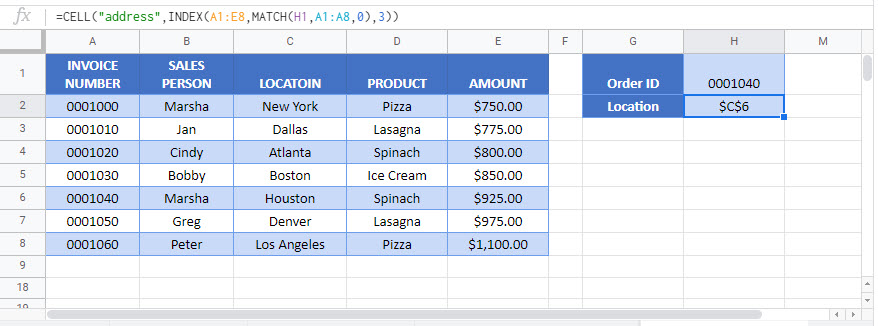This tutorial will demonstrate how to retrieve the address of a looked-up cell instead of the value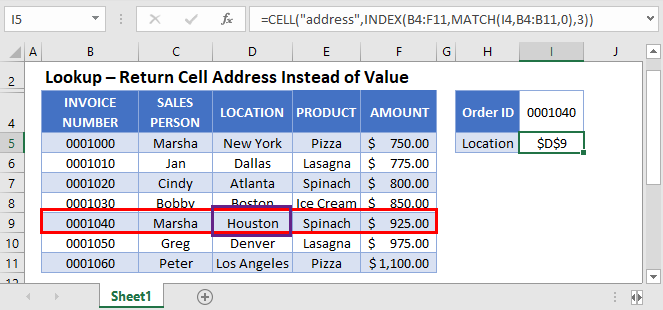To look up a cell address (instead of a cell value), we use the INDEX / MATCH Functions to find the range and the CELL Function to output the cell address:

``=CELL("address",INDEX(B2:F11,MATCH(I2,B2:B11,0),3))``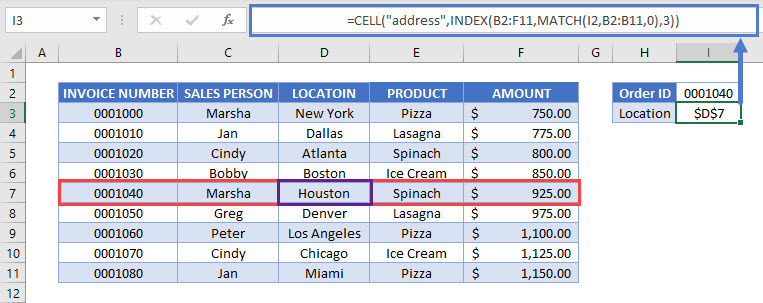We will walk through the formula below.

### MATCH Functions

The MATCH Function looks for the invoice number and returns its row number:

``=MATCH(E2,B2:B11,0)``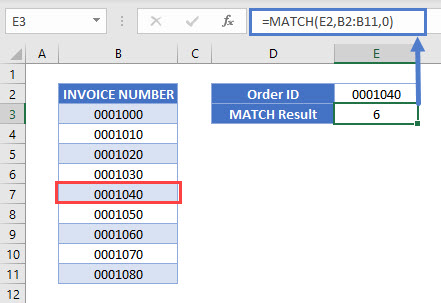## INDEX Function

The INDEX Function returns the cell value corresponding to the row returned by the MATCH Function above:

``=INDEX(B2:F11,MATCH(I2,B2:B11,0),3)``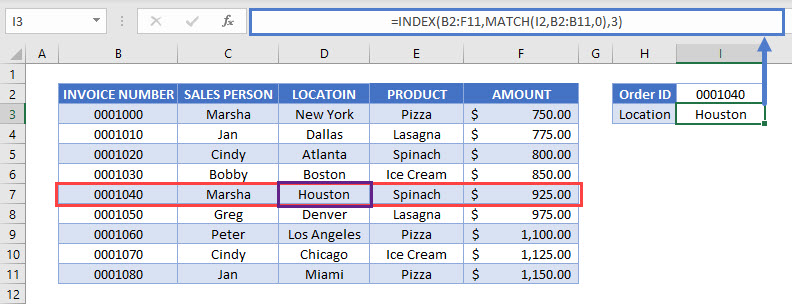### CELL Function – Retrieve Cell Attributes

The CELL Function retrieves specific attributes about a cell. Here we calculate the cell address by inputting “address”:

``=CELL("address",INDEX(B2:F11,MATCH(I2,B2:B11,0),3))``